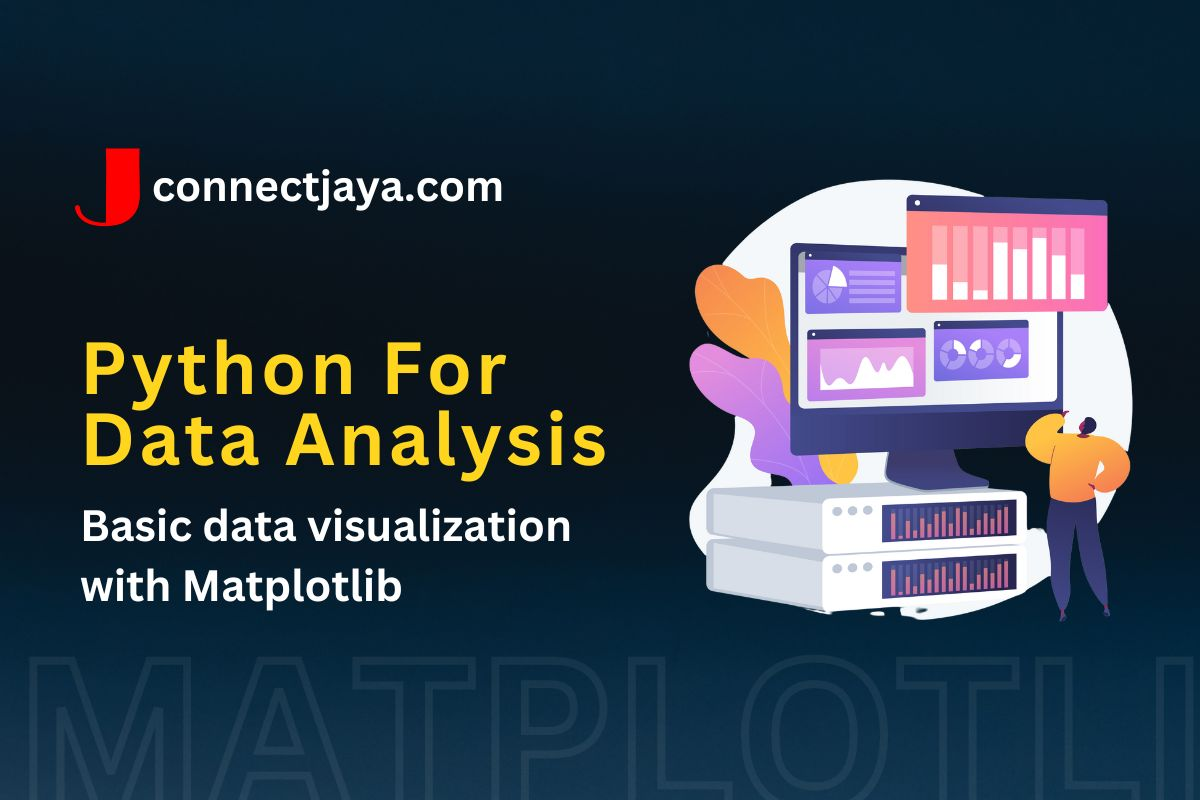## Basic data visualization with Matplotlib

Matplotlib is a powerful data visualization library in Python. It provides a variety of tools for creating a wide range of visualizations, from simple line plots and scatter plots to complex heat maps and contour plots. Here’s an introduction to creating basic data visualizations with Matplotlib.

import matplotlib.pyplot as plt

# create a simple line plot
x = [1, 2, 3, 4, 5]
y = [2, 4, 6, 8, 10]
plt.plot(x, y)
plt.xlabel('x-axis')
plt.ylabel('y-axis')
plt.title('Simple Line Plot')
plt.show()

# create a scatter plot with color-coded points
import numpy as np

x = np.random.randn(100)
y = np.random.randn(100)
colors = np.random.rand(100)
plt.scatter(x, y, c=colors)
plt.xlabel('x-axis')
plt.ylabel('y-axis')
plt.title('Scatter Plot with Color-Coded Points')
plt.show()

# create a bar plot with error bars
x = ['A', 'B', 'C', 'D', 'E']
heights = [10, 20, 30, 40, 50]
errors = [1, 2, 3, 4, 5]
plt.bar(x, heights, yerr=errors)
plt.xlabel('Category')
plt.ylabel('Value')
plt.title('Bar Plot with Error Bars')
plt.show()

# create a stacked bar plot
men_means = [20, 35, 30, 35, 27]
women_means = [25, 32, 34, 20, 25]
ind = np.arange(5)
plt.bar(ind, men_means, label='Men')
plt.bar(ind, women_means, bottom=men_means, label='Women')
plt.xticks(ind, ('G1', 'G2', 'G3', 'G4', 'G5'))
plt.xlabel('Group')
plt.ylabel('Value')
plt.title('Stacked Bar Plot')
plt.legend()
plt.show()


These examples demonstrate the basic syntax for creating line plots, scatter plots, bar plots, and stacked bar plots with matplotlib. By adjusting various parameters and using additional plotting functions provided by Matplotlib, it’s possible to create a wide range of custom visualizations to suit the needs of your data analysis project.

### Use case

Matplotlib can be used for a wide range of data visualization tasks in various domains. Here are some examples of use cases where Matplotlib can be applied:

1. Finance: Visualize stock prices over time using line plots or candlestick charts to identify trends and patterns.
2. Biology: Plot gene expression data using heatmaps or cluster dendrograms to identify genes with similar expression patterns.
3. Social sciences: Create bar charts or pie charts to visualize survey responses or demographic data.
4. Marketing: Use scatter plots or line plots to visualize the relationship between advertising spend and sales revenue.
5. Engineering: Plot sensor data from IoT devices using line plots or scatter plots to identify anomalies or predict failures.
6. Sports analytics: Create box plots or histograms to visualize player performance data or team statistics.

These are just a few examples of the many use cases where Matplotlib can be applied to visualize data in a meaningful way. By combining Matplotlib with other Python libraries such as NumPy and Pandas, it’s possible to create complex and informative visualizations that can help to uncover insights and drive decision-making.